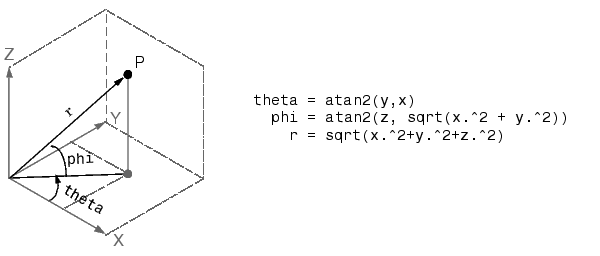MATLAB Function Referencecart2sph

Transform Cartesian coordinates to spherical

Syntax

• ```[THETA,PHI,R] = cart2sph(X,Y,Z)
```

Description

```[THETA,PHI,R] = cart2sph(X,Y,Z) ``` transforms Cartesian coordinates stored in corresponding elements of arrays `X`, `Y`, and `Z` into spherical coordinates. Azimuth `THETA `and elevation `PHI` are angular displacements in radians measured from the positive x-axis, and the x-y plane, respectively; and `R` is the distance from the origin to a point.

Arrays `X`, `Y`, and `Z` must be the same size.

Algorithm

The mapping from three-dimensional Cartesian coordinates to spherical coordinates isSee Also

`cart2pol`, `pol2cart`, `sph2cart`

© 1994-2005 The MathWorks, Inc.